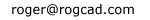```----------------

home

Balloting

Simple trig

Logistic map

The Attractor of Henon

Number doubling

Barnsley's Fern

The Sierpinski Triangle

Barnsley's Fern

A simple four part algorithm, which incorporates
randomly selected parts of the algorithm, produces
a realistic looking fern.  Invented by Michael Barnsley.

Part one of the algorithm is selected 1% of the time.
Part two of the algorithm is selected 85% of the time.
Part three of the algorithm is selected 7% of the time.
Part four of the algorithm is selected 7% of the time.

(See program code further down.)VisualBASIC program code:

Private Sub fernbtn1_Click()

MAG = Val(movebox)
ITER = Val(itera.Text)
yshift = Val(yshiftbox.Text)

Randomize Timer

For a = 1 To ITER

RN = Rnd(1)

If RN < 0.01 Then GoTo 100
If RN < 0.86 Then GoTo 200
If RN < 0.93 Then GoTo 300
GoTo 400

100:
X = 0
Y = 0.16
GoTo 500

200:
X = (0.85 * X) + (0.04 * Y)
Y = (-0.04 * X) + (0.85 * Y + 1.6)
GoTo 500

300:
X = (0.2 * X) - (0.26 * Y)
Y = (0.23 * X + 0.22 * Y + 1.6)
GoTo 500

400:
X = (-0.15 * X) + (0.28 * Y)
Y = (0.26 * X) + (0.24 * Y) + 0.44

500:
TX = (X * 80 * MAG) + 600
TY = (Y * 80 * MAG) + 50 + yshift
viewport.PSet (TX, TY), RGB(26, 125, 0)

Next a

End Sub

======================================================

home

Balloting

Simple trig

Logistic map

The Attractor of Henon

Number doubling

Barnsley's Fern

The Sierpinski Triangle

```